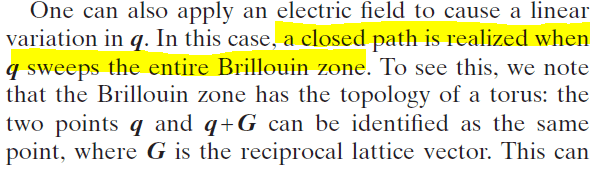# Berry curvature in solids

• A
semc
TL;DR Summary
How is the closed loop evolution formed in solids?This is a paragraph from Rev. Mod. Phys,82,1959(2010). From the article, I understand that Berry phase is gauge invariant only for closed loop evolution but what exactly is this evolution? Does it mean that the system initially start out with some Hamiltonian and I continuously change the Hamiltonian by somehow affecting the parameter of the Hamiltonian? How do I guarantee I go back to the initial Hamiltonian to form the close loop? How is it that by simply applying an electric field to the system I can get a close loop evolution?

Well, the wavefunction of an electron in a periodic potential is of Bloch form: ##\psi_{kn}(x)=u_{kn}(x)\exp(ikx)##, where ##u_{kn}(x)## has the periodicity of the lattice.
The hamiltonian, which has the ##u_{kn}## as eigenfunctions is
##H=\frac{1}{2m}(p-ik)^2+U(x)##, i.e., ##k## appears as a vector potential like term in this effective Hamiltonian.
A spatially constant electric field ##E(t)## can also be formulated as a vector potential ##A(t)=\int^t E(t') dt'.##
So, in the presence of an electric field, ##H(t)=\frac{1}{2m}(p-ik-iA(t))^2+U(x)##.
If the change of A(t) is slow (adiabatic), the eigenfunction ##u_{kn}## gets transformed into ##u_{(k+A(t))n}.##
Now, as for a reciprocal lattice vector G, ##u_{kn}=u_{(k+G)n}##, if ##A(t)=G##, the motion in Hilbert space will have completed a closed loop, up to a phase factor, which contains a Berry part.

•Rzbs and dRic2
semc
I get up until the adiabatic part (I think) but have some problem understanding the last line. Do you mean that if ## A(t)=G ## , then the transformed eigenfunction would be the same as the initial eigenfunction ## u_{kn} ## according to the periodic boundary condition? What is the condition for A(t) to be adiabatic? Sorry I am very new to this.

Yes, the hamiltonian is periodic in k with period G. The question, how slow A(t) has to change with time is difficult and may differ for different states. It will usually hold if ## \langle i | (\partial H(t)/\partial t | j \rangle << | E_i(t)-E_j(t)| ## for all states i and j in question.

semc
Thanks for the clarification. One last question: You said if ##A(t)=G##. When does that happen? Why is ##A(t)=G##?

Thanks for the clarification. One last question: You said if ##A(t)=G##. When does that happen? Why is ##A(t)=G##?

With a constant electric field ##E##, ##A(t) \sim Et##, so ##A## will be periodically equal to a reciprocal lattice vector ##G##.

semc
Sorry I don't see why A is periodic in k-space if it does not have spatial dependence•